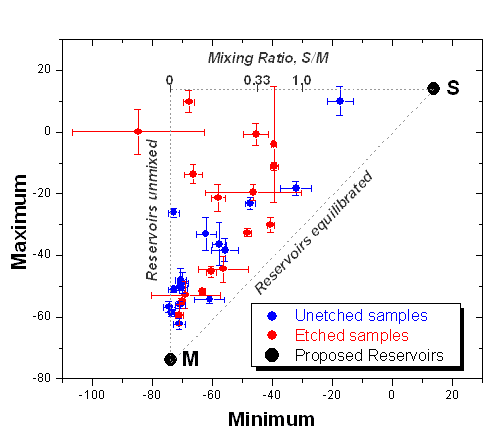Skip Navigation Links.

### Scatter Plot Depicting Isotope Signature of N Sources Found in IAB Iron MeteoritesThe graph shown here depicts the isotope signature of N sources found in IAB iron meteorites. It was made by adding a series of plots, one on top of another, as follows:

1. First, the sample data was plotted as a Scatter plot with X and Y error bars. During plotting, data labels were included with each individual meteorite. In addition, the color was indexed to distinguish between etched and un-etched samples.
2. Next, the two proposed N sources were introduced as a small Scatter plot with data labels.
3. Then, a bounding triangle was added as a simple dotted Line plot.
4. A Scatter plot of the mixing ratios for the two proposed sources was added on the top of the triangle-shaped Line plot. Data labels were included along with the plot.
5. Labels explaining the three sides of the triangle were inserted.
6. Finally a legend was added to explain the three types of data points in the plot, adjusting the legend text color to reflect the color of specific data points.

Background

Dr. K. Poganis is a staff research associate in cosmochemistry in the Chemistry and Biochemistry department at UCSD. Cosmochemistry is stable isotope chemistry, or the study of extra-terrestrial material, like meteorites or lunar samples. The department uses a special type of static mass spectrometer for their measurements.